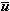﻿ An Amuse-Bouche from APL History

An Amuse-Bouche from APL History

Roger K.W. Hui

The phrase x[⍋⍒(⍴x)⍴0 1] appeared on the back of I.P. Sharp T-shirts in the 1970s and 1980s. It performs a perfect shuffle.

```   x←'ABCDEFGHIJKLMNOPQ'
x[⍋⍒(⍴x)⍴0 1]
IAJBKCLDMENFOGPHQ
```

I was curious about its provenance and found the following:

Smith, Robert A., Problem Section, APL Quote Quad, Volume 3, Number 1, 1971-06-11, page 21.

 2: Mesh Problem (K.E. Iverson): Given vectors a , b and p such that a and b are either both numeric or both character and ``` p∊ 0 1 (⍴a)=+/~p (⍴b)=+/p ``` Write an APL expression whose value, in either origin, is the vector obtained by storing consecutive values left to right of a into the 0’s of p and b into the 1’s of p . For example: ``` a←'O JS' b←'TMONE' p←1 0 1 0 0 1 1 1 0 ``` yields   TOM JONES There are two known minimal solutions. (cf. Algorithm 48, APL ⍞, Vol. 11 No. 6).

Robert A. Smith of Laurel, Maryland was the editor of the problem section in the APL Quote Quad. He is now better known as Bob Smith of Boolean Bob, NARS, NARS2000, and 386MAX (and other) fame.

Mesh was described in §1.9 of A Programming Language, 1962:

 A logical vector u and the two vectors a =/c and b = u/c, obtained by compressing a vector c, collectively determine the vector c. The operation which specifies c as a function of a, b, and u is called a mesh and is defined as follows: If a and b are arbitrary vectors and if u is a logical vector such that +/= ν(a) and +/u = ν(b), then the mesh of a and b on u is denoted by \a, u, b\ and is defined as the vector c such that/c = a and u/c = b. The mesh operation is equivalent to choosing successive components of c from a or b according as the successive components of u are 0 or 1. If, for example, a = (s, e, k), b = (t, a), and u = (0, 1, 0, 1, 0), then \a, u, b\ = (s, t, e, a, k). As a further example, …

Algorithm 48, entitled “Merging”, was a 13-line branching and looping function submitted by James H. Burrill and Clark Wiedmann. The 13 lines included one comment line and three error-checking lines. There was an additional note that the function can be used to merge, rearrange, or rotate vectors.

In the immediately following issue of the APL Quote Quad, Volume 3, Number 2 & 3, 1971-10-01, there were two relevant articles. The first (page 52) was Algorithm 67 submitted by H.J. Myers of the IBM San Jose Programming Center.

 ``` ∇ z←a merge b  ⍝Algorithm 48a  →((⍴a←,a)>⍴b←,b)/e1  z←b[⍋⍋a]  →0  e1:'length error' ∇ ```

The second (page 61) was solution 2 in the problem section:

 2.  (a,b)[⍋⍋p] or (b,a)[⍋⍒p] . This problem were incorrectly attributed to Ken Iverson who has since informed me that he learned of it from Luthor Woodrum. It illustrates a beautiful and succinct use of the sort operators. Note the interesting relationship between the two solutions. Using this idea, Algorithm 48 in Vol. 11, No. 6 of this magazine can be shortened to ``` ∇ z←a merge b  →(0⍴a=b),0⍴∧/a  z←b[⍋⍒a] ∇ ```

From the above, we can say that the mesh problem was due to Ken Iverson and the solution to Luthor Woodrum and perhaps to H.J. (Joseph) Myers.

More recently, x[⍋⍒(⍴x)⍴0 1] is the subject of a Vector article by Norman Thomson (2005-05) and a D-function page by John Scholes (2007-03-05), and is item 6 of Sixteen APL Amuse-Bouches by myself (2014-10-27).

In Dyalog APL, mesh can be implemented as a monadic operator:

```   M←{(⍺,⍵)[⍋⍋⍺⍺]}   ⍝ or M←{(⍵,⍺)[⍋⍒⍺⍺]}

'O JS' (1 0 1 0 0 1 1 1 0 M) 'TMONE'
TOM JONES
```

The “selfie” x(b M)x or b M⍨ x (same left and right arguments) is equivalent to the merge functions described above. Moreover:

```   1 0 1 1 1 1 1 M⍨ ⍳7
1 0 2 3 4 5 6
1 1 1 1 1 1 0 M⍨ ⍳7
1 2 3 4 5 6 0
```

These two permutations, p←(⌽⍳2⌊n),2↓⍳n and q←1⌽⍳n , suffice to generate the entire group of permutations of order n (see §4.4 of my APL87 paper or exercise 2.10.11 of Herstein’s Topics in Algebra). Therefore, repeated application of M with left arguments 1≠⍳n and n≠1+⍳n suffice to effect any permutation of a vector of length n .

 created: 2014-10-25 09:10 updated: 2014-10-28 09:45